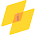• ## How to Create Effortless Diagrams in Simulink

MATLAB Simulink from Basics

• ## How to export Simulink Graph data into MATLAB and Excel

1.I have an error

2.I have an error

1.What is the error?? Tell us

3.I need NURBS Surface heat transfer problem by collocation method MATLAB program plz help me

1.Please mail us your complete problems: castorclasses2014@gmail.com

4.I NEED EXPLANATION FOR THE BELOW CODE

[r,c,n] = size(img);
if n > 1
im=rgb2gray(img);
else
im=img;
end

tic,
a=1;
figure,imshow(img), title('processing Image')
for j=1:overlapp:(c-mtx_cal)+1
for i=1:overlapp:(r-mtx_cal)+1

sondos(a).block=im(i:i+mtx_cal-1,j:j+mtx_cal-1);
sondos(a).position=[i j];
sondos(a).index=a;
a=a+1;
end
end

5.bus2=[10,0.2,60];
bus3=[20,0.5,50];
for i=1:3
filename = ['bus', num2str(i)];
x(i)=filename(3);
FE(i)= abs(50.23333-filename(3));
End
for each iteration filname becomes bus1, bus2 and bus3 then I want to make each values of filename again variable sothat bus1(3) = 50
the answer that I want to be as follows
X=
50
60
50
FE=
0.23333
10.23333
0.23333

6.nutrAttractive is a scientifically formulated, complete health, beauty & immunity solution with an unprecedented combination of 50 potent clinically proven and highly bio-available ingredients, that functions at the cellular level to make your body “Healthy Inside and Attractive Outside”.click>

MATLAB